# STL源码剖析-vector

2019/03/21 21:36

## vector

 1 #include <iostream>
2 #include <vector>
3 #include <algorithm>
4 using namespace std;
5
6 // unique_ptr::get vs unique_ptr::release
7 int main()
8 {
9     //初始化
10     vector<int> vec;//声明，未初始化
11     vector<int> vec1(2, 5);//2个5
12     vector<int> vec2 = { 1, 2, 3, 4, 5 };//直接初始化
13     //读取元素
14     cout << "第2个元素: " << vec2 << endl;
15     cout << "首元素: " << vec2.front() << endl;
16     cout << "尾元素: " << vec2.back() << endl;
17     //插入元素
18     vec1.insert(vec1.begin(), 999);//para1-插入位置，要迭代器即指针，para2-插入内容
19     vec2.push_back(888);//尾部插入
20     vec2.pop_back();//删除尾部，类似于stack，所以有时候也可以把vector当stack用
21     //删除元素
22     vec2.erase(vec2.end()-1);//参数也是迭代器类型，所以使用insert和erase时，最好用iterator来遍历
23     //排序
24     sort(vec2.begin(), vec2.end());//给出首位指针
25     sort(vec2.begin(), vec2.begin()+3);//甚至这样也可以，因为iterator本身就是类型指针
26
27     //遍历--下标索引访问
28     cout << "vec1 : " ;
29     for (int i = 0; i < vec1.size(); ++i)
30     {
31         cout << vec1[i] << " ";
32     }
33
34     //遍历--迭代器指针访问
35     cout << "\nvec2 : ";
36     vector<int>::iterator it;
37     for (it=vec2.begin(); it != vec2.end(); ++it)
38     {
39         cout << *it << " ";
40     }41　　　//使用指针遍历vector　　　　auto vec = new vector<int>(10, 8);　　　　for(int i=0; i<vec->size(); i++)　　　　　　cout << (*vec)[i] << "  ";
41     return 0;
42 }

### 底层是怎么实现的呢？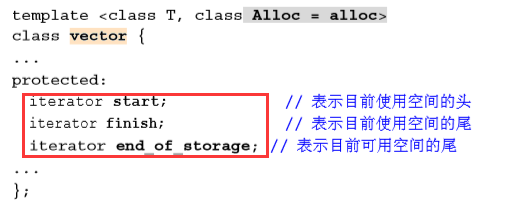### 库函数的实现呢？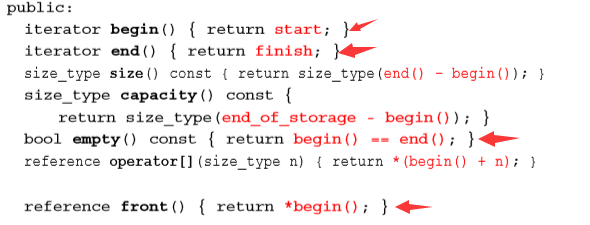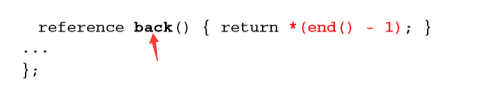### 那么我们再来探讨一下，有意思的东西。

插入元素时，预设的end_of_storage不够怎么办？怎么进行扩容。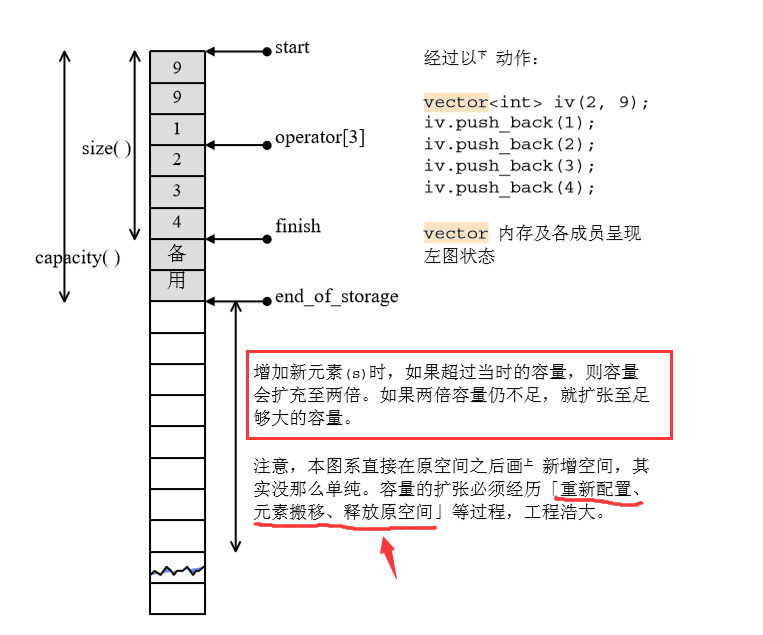### 再看源码！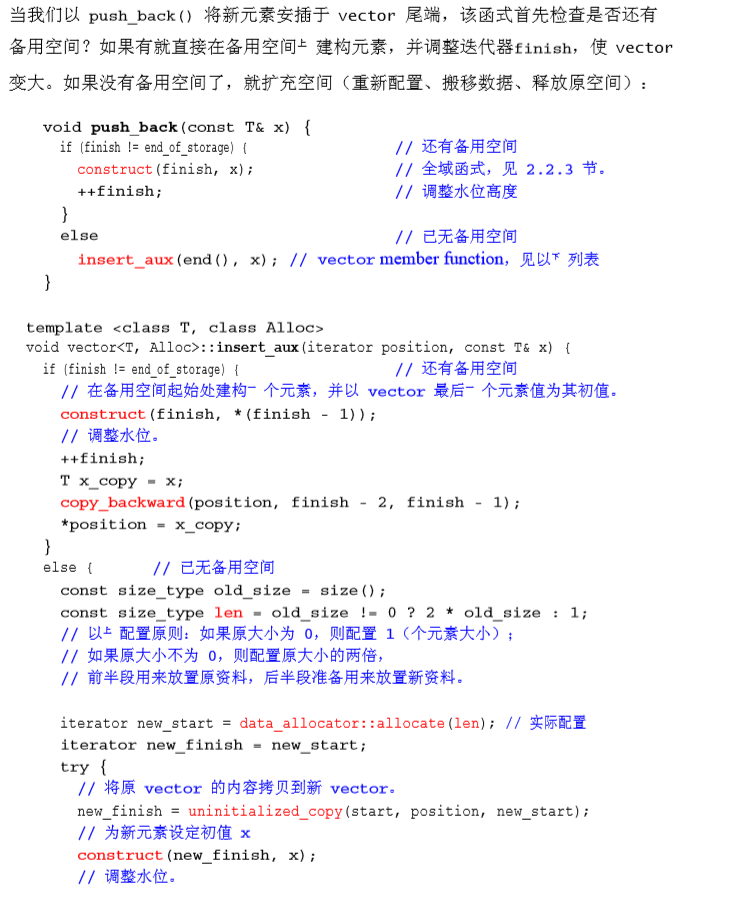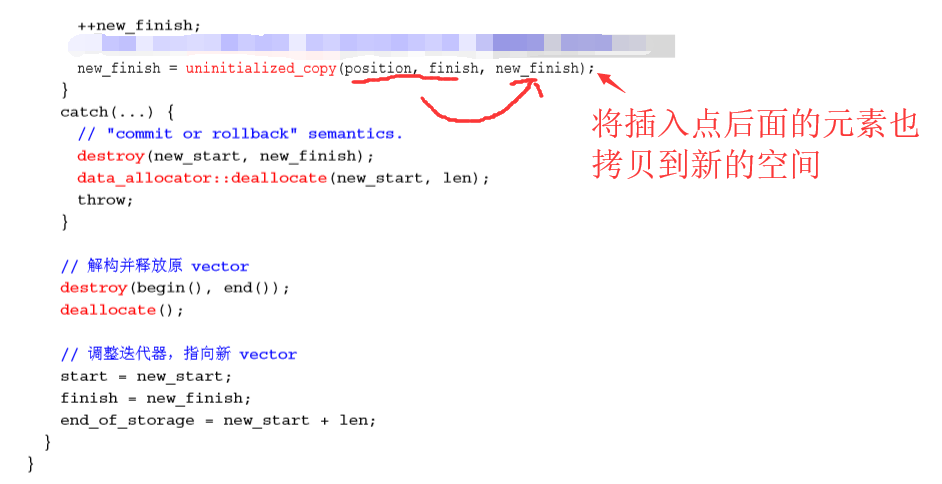1.先申请两倍内存，判断够不够，够进入2；否则，分配需要的大小；

2.拷贝要插入点之前的内容

3.构造插入元素顺次添加到后面

4.接着把之前插入点后面的内容拷贝到新的空间中

5.释放原来空间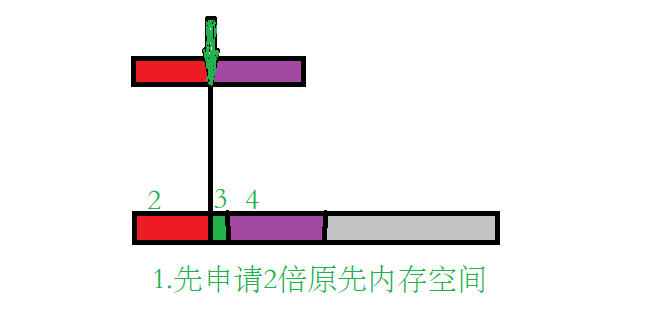1 int main()
2 {
3     vector<int> vec;
4     for(int i=0; i<20; i++)
5     {
6         cout << "vector size= " << vec.size() << endl;
7         cout << "vector capacity= " << vec.capacity() << endl;
8         //cout << "vector max_size= " << vec.max_size() << endl;
9         vec.push_back(i);
10     }
11     cout << "最后一次, 插入100个元素 " << endl;
12     vec.insert(vec.begin(), 100, 1);
13     cout << "vector size= " << vec.size() << endl;
14     cout << "vector capacity= " << vec.capacity() << endl;
15     //cout << "vector max_size= " << vec.max_size() << endl;
16
17     return 0;
18 }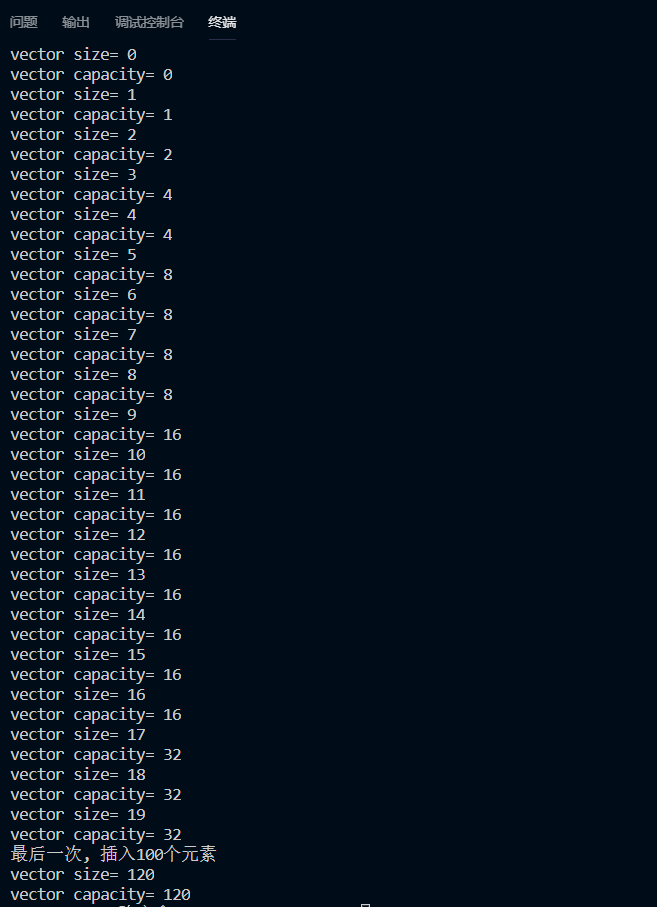1 int main()
2 {
3     vector<int> vec;
4     for(int i=0; i<20; i++)
5     {
6         cout << "Max_size = " << vec.max_size() << endl;
7         cout << "size = " << vec.size() << endl;
8         vec.push_back(i);
9         cout << "插入 " << i << endl;
10     }
11     return 0;
12 }

（1）GCC 1019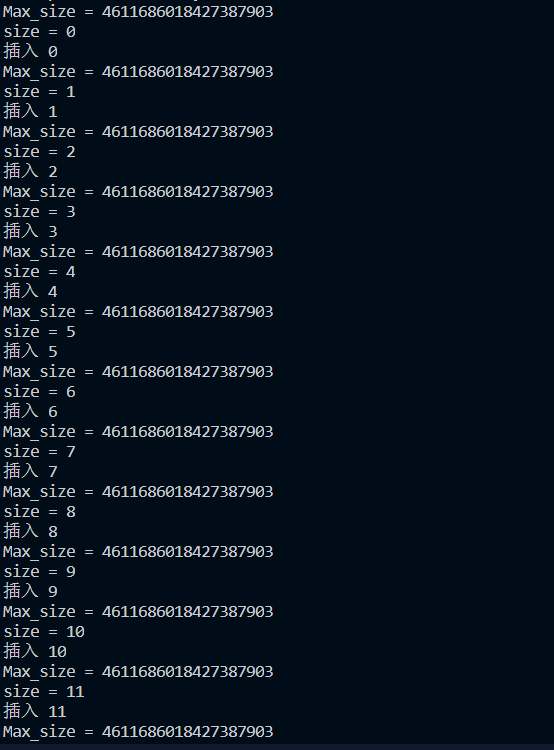（2）MSVC 109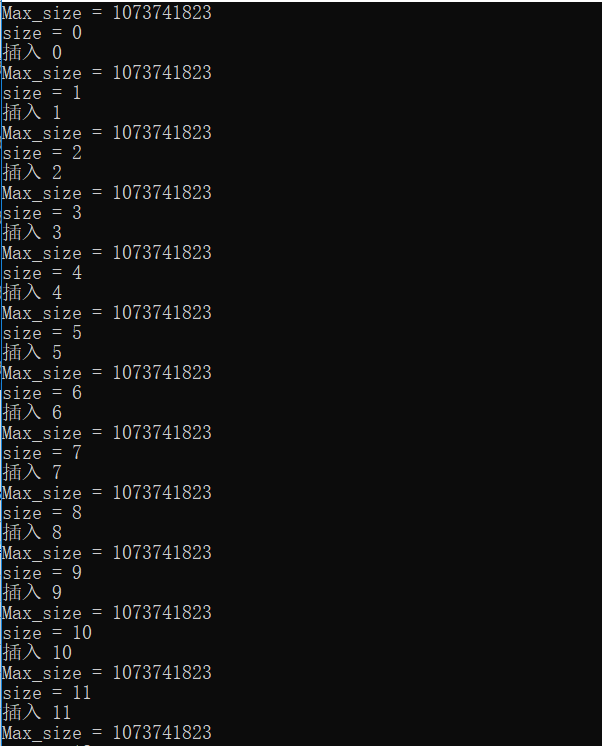代码来自《STL源码解析》，源码迸发着光辉！

0
0 收藏

### 作者的其它热门文章0 评论
0 收藏
0